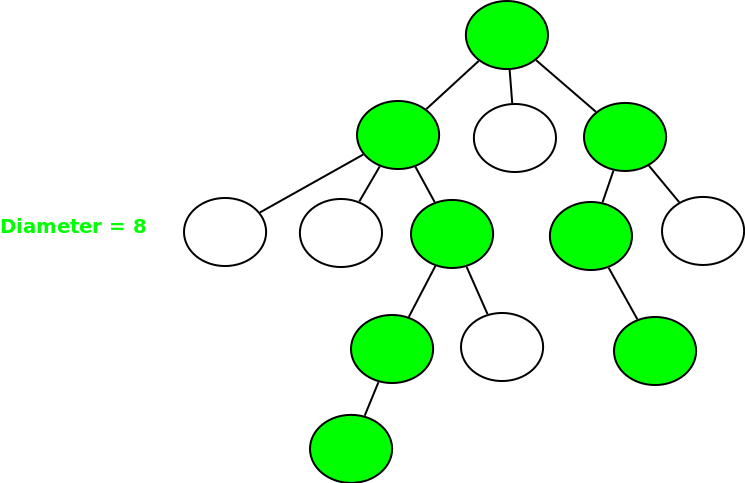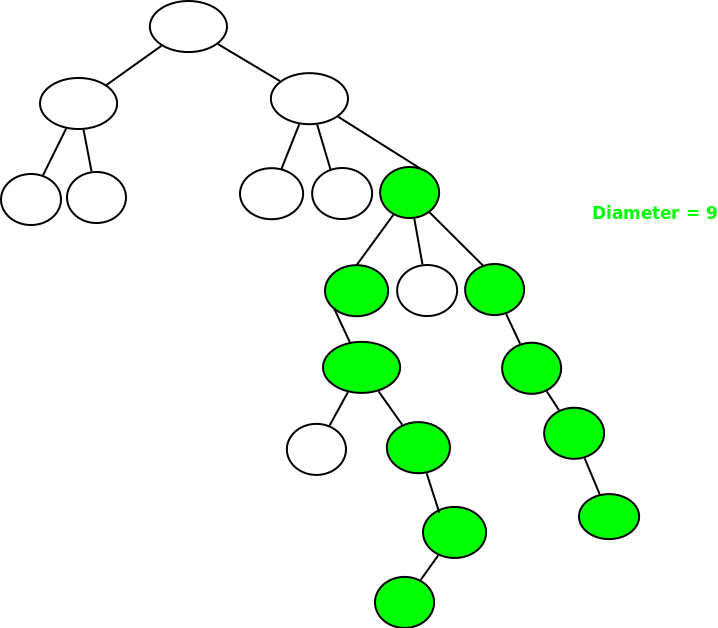Related Articles
DP on Trees | Set-3 ( Diameter of N-ary Tree )
• Difficulty Level : Hard
• Last Updated : 14 Feb, 2019

Given an N-ary tree T of N nodes, the task is to calculate the longest path between any two nodes(also known as the diameter of the tree).

Example 1:Example 2:## Recommended: Please try your approach on {IDE} first, before moving on to the solution.

Different approaches to solve these problems have already been discussed:

In this post, we will be discussing an approach which uses Dynamic Programming on Trees.

Prerequisites:

There are two possibilities for the diameter to exist:

• Case 1: Suppose the diameter starts from a node and ends at some node in its subtree. Let’s say that there exist a node x such that the longest path starts from node x and goes into its subtree and ends at some node in the subtree itself. Let’s define this path length by dp1[x].
• Case 2: Suppose the diameter or the longest path starts in subtree of a node x, passes through it and ends in it’s subtree. Let’s define this path by dp2[x].

If for all nodes x, we take a maximum of dp1[x], dp2[x], then we will get the diameter of the tree.

For the case-1, to find dp1[node], we need to find the maximum of all dp1[x], where x is the children of node. And dp1[node] will be equal to 1 + max(dp1[children1], dp1[children2], ..).

For the case-2, to find dp2[node], we need to find the two maximum of all dp1[x], where x is the children of node. And dp2[node] will be equal to 1 + max 2 of(dp1[children1], dp1[children2], ..).

We can easily run a DFS and find the maximum of both dp1[node] and dp2[node] for every to get the diameter of the tree.

Below is the implementation of the above approach:

## C++

 `// C++ program to find diameter of a tree ` `// using DFS. ` `#include ` `using` `namespace` `std; ` ` `  `int` `diameter = -1; ` ` `  `// Function to find the diameter of the tree ` `// using Dynamic Programming ` `int` `dfs(``int` `node, ``int` `parent, ``int` `dp1[], ``int` `dp2[], list<``int``>* adj) ` `{ ` ` `  `    ``// Store the first maximum and secondmax ` `    ``int` `firstmax = -1; ` `    ``int` `secondmax = -1; ` ` `  `    ``// Traverse for all children of node ` `    ``for` `(``auto` `i = adj[node].begin(); i != adj[node].end(); ++i) { ` `        ``if` `(*i == parent) ` `            ``continue``; ` ` `  `        ``// Call DFS function again ` `        ``dfs(*i, node, dp1, dp2, adj); ` ` `  `        ``// Find first max ` `        ``if` `(firstmax == -1) { ` `            ``firstmax = dp1[*i]; ` `        ``} ` `        ``else` `if` `(dp1[*i] >= firstmax) ``// Secondmaximum ` `        ``{ ` `            ``secondmax = firstmax; ` `            ``firstmax = dp1[*i]; ` `        ``} ` `        ``else` `if` `(dp1[*i] > secondmax) ``// Find secondmaximum ` `        ``{ ` `            ``secondmax = dp1[*i]; ` `        ``} ` `    ``} ` ` `  `    ``// Base case for every node ` `    ``dp1[node] = 1; ` `    ``if` `(firstmax != -1) ``// Add ` `        ``dp1[node] += firstmax; ` ` `  `    ``// Find dp ` `    ``if` `(secondmax != -1) ` `        ``dp2[node] = 1 + firstmax + secondmax; ` ` `  `    ``// Return maximum of both ` `    ``return` `max(dp1[node], dp2[node]); ` `} ` ` `  `// Driver Code ` `int` `main() ` `{ ` `    ``int` `n = 5; ` ` `  `    ``/* Constructed tree is  ` `         ``1  ` `        ``/ \  ` `        ``2 3  ` `       ``/ \  ` `       ``4  5 */` `    ``list<``int``>* adj = ``new` `list<``int``>[n + 1]; ` ` `  `    ``/*create undirected edges */` `    ``adj.push_back(2); ` `    ``adj.push_back(1); ` `    ``adj.push_back(3); ` `    ``adj.push_back(1); ` `    ``adj.push_back(4); ` `    ``adj.push_back(2); ` `    ``adj.push_back(5); ` `    ``adj.push_back(2); ` ` `  `    ``int` `dp1[n + 1], dp2[n + 1]; ` `    ``memset``(dp1, 0, ``sizeof` `dp1); ` `    ``memset``(dp2, 0, ``sizeof` `dp2); ` ` `  `    ``// Find diameter by calling function ` `    ``cout << ``"Diameter of the given tree is "` `         ``<< dfs(1, 1, dp1, dp2, adj) << endl; ` ` `  `    ``return` `0; ` `} `

## Python3

 `# Python3 program to find diameter  ` `# of a tree using DFS.  ` ` `  `# Function to find the diameter of the  ` `# tree using Dynamic Programming  ` `def` `dfs(node, parent, dp1, dp2, adj):  ` ` `  `    ``# Store the first maximum and secondmax  ` `    ``firstmax, secondmax ``=` `-``1``, ``-``1` ` `  `    ``# Traverse for all children of node  ` `    ``for` `i ``in` `adj[node]:  ` `        ``if` `i ``=``=` `parent:  ` `            ``continue` ` `  `        ``# Call DFS function again  ` `        ``dfs(i, node, dp1, dp2, adj)  ` ` `  `        ``# Find first max  ` `        ``if` `firstmax ``=``=` `-``1``:  ` `            ``firstmax ``=` `dp1[i]  ` `         `  `        ``elif` `dp1[i] >``=` `firstmax: ``# Secondmaximum  ` `            ``secondmax ``=` `firstmax  ` `            ``firstmax ``=` `dp1[i]  ` `         `  `        ``elif` `dp1[i] > secondmax: ``# Find secondmaximum  ` `            ``secondmax ``=` `dp1[i]  ` ` `  `    ``# Base case for every node  ` `    ``dp1[node] ``=` `1` `    ``if` `firstmax !``=` `-``1``: ``# Add  ` `        ``dp1[node] ``+``=` `firstmax  ` ` `  `    ``# Find dp  ` `    ``if` `secondmax !``=` `-``1``: ` `        ``dp2[node] ``=` `1` `+` `firstmax ``+` `secondmax  ` ` `  `    ``# Return maximum of both  ` `    ``return` `max``(dp1[node], dp2[node])  ` ` `  `# Driver Code  ` `if` `__name__ ``=``=` `"__main__"``: ` ` `  `    ``n, diameter ``=` `5``, ``-``1` ` `  `    ``adj ``=` `[[] ``for` `i ``in` `range``(n ``+` `1``)] ` `     `  `    ``# create undirected edges ` `    ``adj[``1``].append(``2``)  ` `    ``adj[``2``].append(``1``)  ` `    ``adj[``1``].append(``3``)  ` `    ``adj[``3``].append(``1``)  ` `    ``adj[``2``].append(``4``)  ` `    ``adj[``4``].append(``2``)  ` `    ``adj[``2``].append(``5``)  ` `    ``adj[``5``].append(``2``)  ` ` `  `    ``dp1 ``=` `[``0``] ``*` `(n ``+` `1``)  ` `    ``dp2 ``=` `[``0``] ``*` `(n ``+` `1``)  ` `     `  `    ``# Find diameter by calling function  ` `    ``print``(``"Diameter of the given tree is"``, ` `                 ``dfs(``1``, ``1``, dp1, dp2, adj)) ` ` `  `# This code is contributed by Rituraj Jain  `

Output:

```Diameter of the given tree is 4
```

Attention reader! Don’t stop learning now. Get hold of all the important DSA concepts with the DSA Self Paced Course at a student-friendly price and become industry ready.

My Personal Notes arrow_drop_up
Recommended Articles
Page :